# pyvista.plotting.charts.ScatterPlot2D#

class ScatterPlot2D(chart, x, y, color='b', size=10, style='o', label='')[source]#

Class representing a 2D scatter plot.

Users should typically not directly create new plot instances, but use the dedicated 2D chart’s plotting methods.

Parameters:
chart`Chart2D`

The chart containing this plot.

xarray_like

X coordinates of the points to draw.

yarray_like

Y coordinates of the points to draw.

color`ColorLike`, default: “b”

Color of the points drawn in this plot. Any color parsable by `pyvista.Color` is allowed.

size`float`, default: 10

Size of the point markers drawn in this plot.

style`str`, default: “o”

Style of the point markers drawn in this plot. See ScatterPlot2D.MARKER_STYLES for a list of allowed marker styles.

label`str`, default: “”

Label of this plot, as shown in the chart’s legend.

Notes

MARKER_STYLESdict

Dictionary containing all allowed marker styles as its keys.

Marker styles#

Style

Description

Example

`""`

Hidden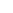`"x"`

Cross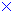`"+"`

Plus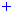`"s"`

Square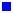`"o"`

Circle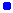`"d"`

Diamond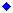Examples

Plot a simple sine wave as a scatter plot.

```>>> import pyvista
>>> import numpy as np
>>> x = np.linspace(0, 2*np.pi, 20)
>>> y = np.sin(x)
>>> chart = pyvista.Chart2D()
>>> _ = chart.scatter(x, y)
>>> chart.show()
```

Methods

 Toggle the plot's visibility. Update this plot's points.

Attributes

 `ScatterPlot2D.brush` Brush object controlling how shapes in this plot are filled. `ScatterPlot2D.color` Return or set the plot's color. `ScatterPlot2D.label` Return or set the this plot's label, as shown in the chart's legend. `ScatterPlot2D.line_style` Return or set the line style of all lines drawn in this plot. `ScatterPlot2D.line_width` Return or set the line width of all lines drawn in this plot. `ScatterPlot2D.marker_size` Return or set the plot's marker size. `ScatterPlot2D.marker_style` Return or set the plot's marker style. `ScatterPlot2D.pen` Pen object controlling how lines in this plot are drawn. `ScatterPlot2D.visible` Return or set the this plot's visibility. `ScatterPlot2D.x` Retrieve the X coordinates of this plot's points. `ScatterPlot2D.y` Retrieve the Y coordinates of this plot's points.